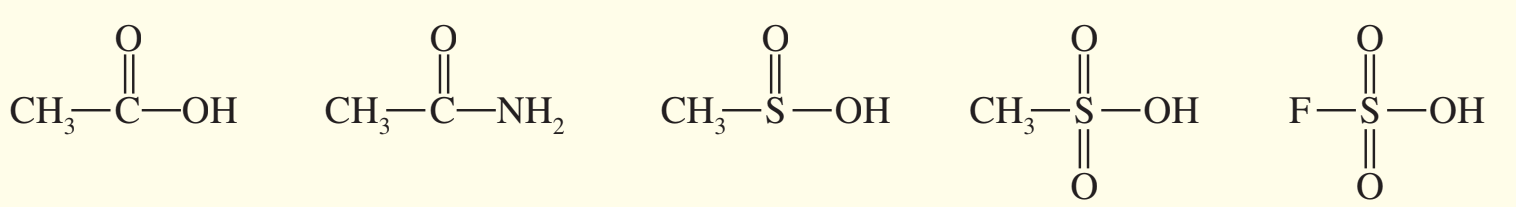×
Get Full Access to Organic Chemistry - 8 Edition - Chapter 1 - Problem 52sp
Get Full Access to Organic Chemistry - 8 Edition - Chapter 1 - Problem 52sp

×

# Solved: The following compounds can all react as acids.ISBN: 9780321768414 33

## Solution for problem 52SP Chapter 1

Organic Chemistry | 8th Edition

• Textbook Solutions
• 2901 Step-by-step solutions solved by professors and subject experts
• Get 24/7 help from StudySoup virtual teaching assistantsOrganic Chemistry | 8th Edition

4 5 1 274 Reviews
25
4
Problem 52SP

The following compounds can all react as acids.(a) For each compound, show its conjugate base. Show any resonance forms if applicable.

(b) Rank the conjugate bases in the order you would predict, from most stable to least stable.

(c) Rank the original compounds in order, from strongest acid to weakest acid.

Equation Transcription:Text Transcription:

CH3-C-OH

CH3-C-NH2

CH3-S-OH

CH2-S-OH

F-S-OH

Step-by-Step Solution:

Solution 52SP :

Step 1:

(a) For each compound, show its conjugate base. Show any resonance forms if applicable.

• A resonance form is another way of drawing a Lewis dot structure for a compound when there is more than one possibility of placing valence or lone pairs of electrons, but the atoms in the resonance forms should have identical position. Equivalent Lewis structures of same compound are called resonance forms.
• To find the conjugate base of an acid, we remove one hydrogen atom and decrease the charge of the compound by 1.

Step 2 of 4

Step 3 of 4

##### ISBN: 9780321768414

Organic Chemistry was written by and is associated to the ISBN: 9780321768414. The full step-by-step solution to problem: 52SP from chapter: 1 was answered by , our top Chemistry solution expert on 05/06/17, 06:41PM. The answer to “?The following compounds can all react as acids. (a) For each compound, show its conjugate base. Show any resonance forms if applicable.(b) Rank the conjugate bases in the order you would predict, from most stable to least stable.(c) Rank the original compounds in order, from strongest acid to weakest acid.Equation Transcription: Text Transcription:CH3-C-OH CH3-C-NH2CH3-S-OHCH2-S-OHF-S-OH” is broken down into a number of easy to follow steps, and 54 words. This full solution covers the following key subjects: acid, stable, show, Rank, order. This expansive textbook survival guide covers 25 chapters, and 1336 solutions. This textbook survival guide was created for the textbook: Organic Chemistry, edition: 8. Since the solution to 52SP from 1 chapter was answered, more than 2097 students have viewed the full step-by-step answer.

## Discover and learn what students are asking

Chemistry: The Molecular Nature of Matter and Change : Kinetics: Rates and Mechanisms of Chemical Reactions
Assume water boils at 100.0?C in Houston (near sea level), and at 90.0?C in Cripple Creek, Colorado (near 9500 ft). If it takes 4.8 min to cook an egg

Statistics: Informed Decisions Using Data : The Randomized Complete Block Design
?Given the following ANOVA output, answer the questions that follow: (a) The researcher wants to test H0: m1 = m2 = m3 =

Unlock Textbook Solution